# How to generate random weekday or weekend date in Excel?

This tutorial is designed to help learners to understand the technique to generate random weekday or weekend date in excel. In this tutorial, the user will understand three possible methods to perform this task. First method is based on using predefined formula, to generate random date. Second method allow user to use kutool to generate the random dates, and last method allow user to use the VBA code to generate the date randomly. Same method can be used to generate both weekday and weekend dates.

## Example 1: Example to generate random weekday date in excel.

### Step 1:

To understand the guided step, firstly create an excel sheet with below given table data: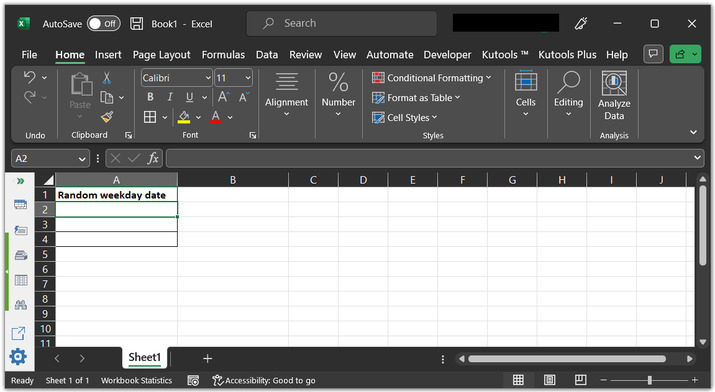### Step 2:

After that go to A2 cell. Type the provided formula, “=DATE(2023, 4, 1)+LARGE(IF(WEEKDAY(DATE(2023, 4, 1)+ROW($1:$365)-1, 2)<6, ROW($1:$365)-1, ""), RANDBETWEEN(1, SUM(--(WEEKDAY(DATE(2023, 1, 1)+ROW($1:$365)-1, 2)<6))))” to the cell.

### Explanation

DATE() is a predefined excel method to generate the date without any need to define a method. Simply, call the method, and pass the required parameters to generate a date.

WEEKDAY() − this method will generate a date lies in the weekday.

2023, is the current taken year.

SUM(): it is a method used to calculate sum.

### Step 3:

Press “Enter” key. This will display a random weekday, as depicted below: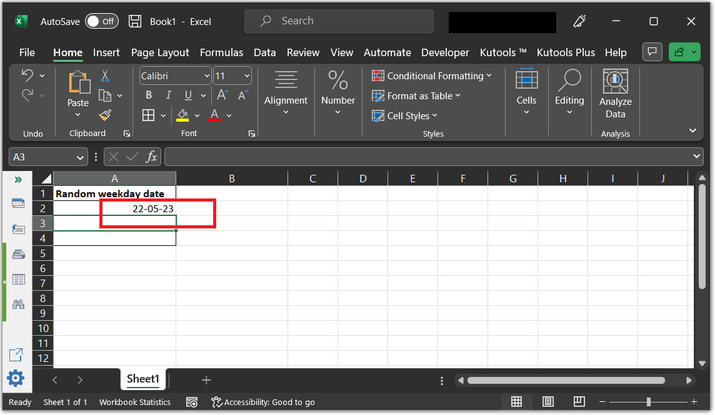## Example 2: Example to generate random weekend date in excel by using the kutool.

### Step 1:

Consider below given sample worksheet with A2, active cell.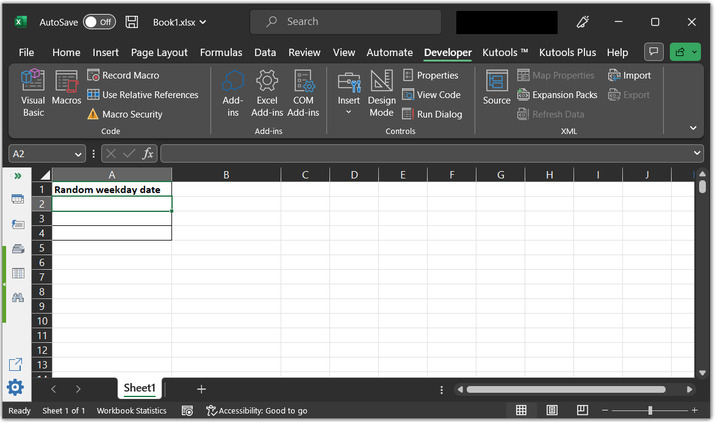### Step 2:

After that go to the “Kutools” tab and choose “Editing” option. Further select the “Insert” option. From the newly appeared drop-down menu simply choose “Insert Random Data”.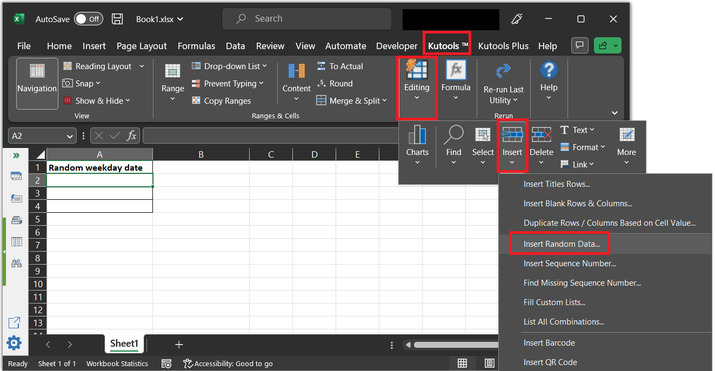### Step 3:

This will open the below given “Insert Random Data” dialog box.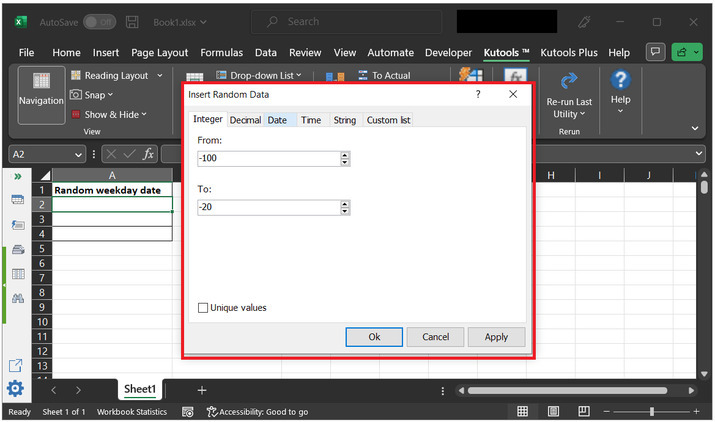### Step 4:

Select the “Date” tab and set the date in from and to input drop-down menu. Select “Workday” if user wants to generate a weekday. For this case, let’s generate a “weekend” date. Finally, click on “OK”.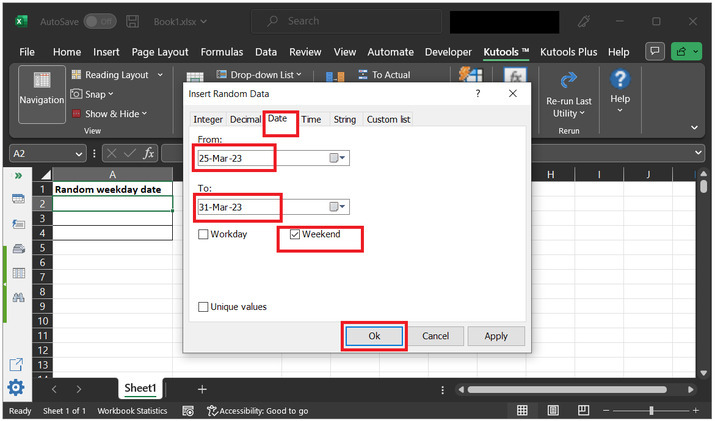### Step 5:

This will display a random date on console.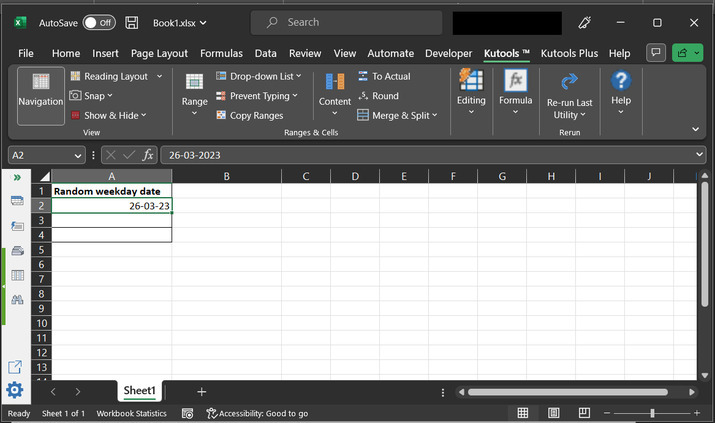## Example 3: Example to generate random weekday date in excel by using VBA code.

### Step 1:

Consider below given sample worksheet.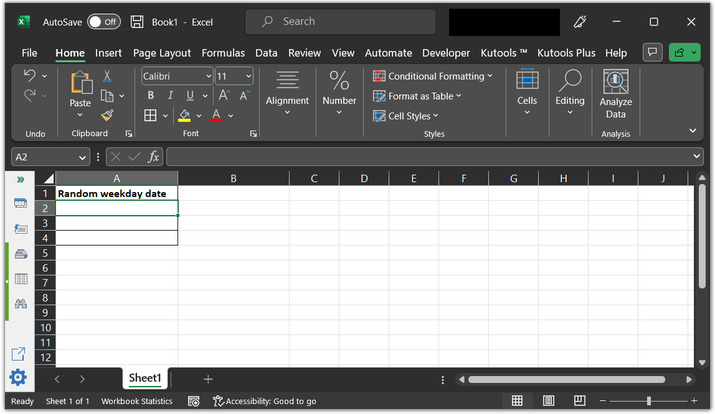### Step 2:

After that go to the “Developer” tab, under the “code” section select the “Visual Basic” code.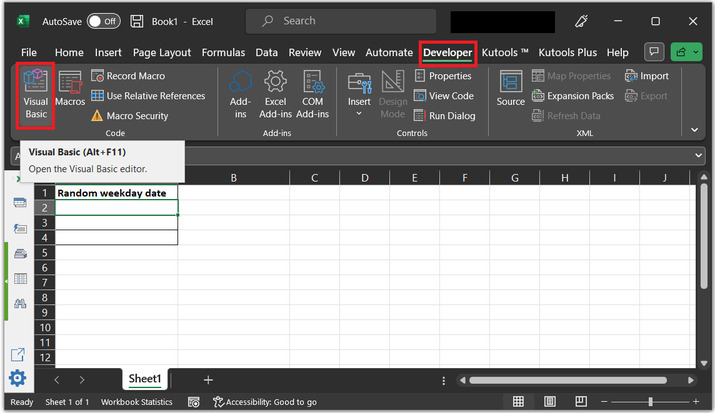### Step 3:

This will open the below given code area: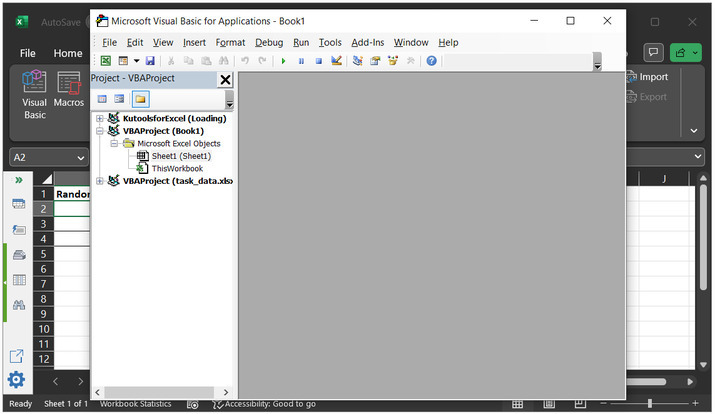### Step 4:

After that go to insert tab and click on “Module”.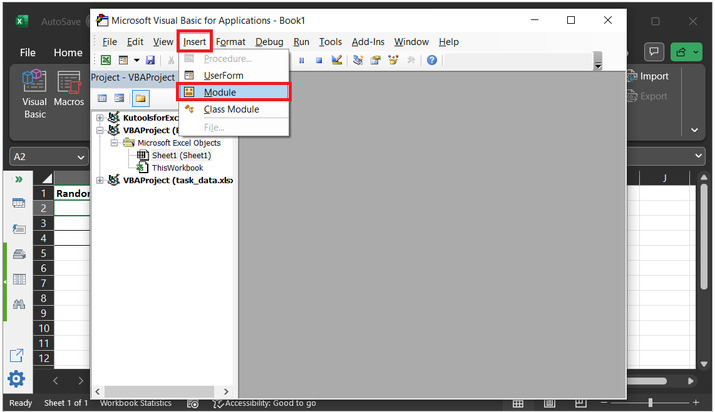### Step 5:

This will open an area to write module code.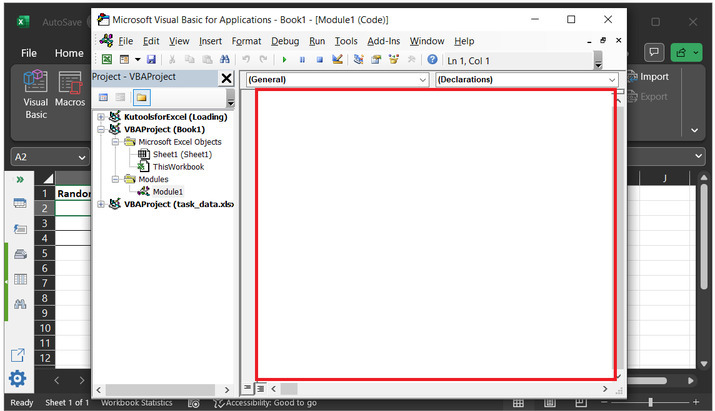### Step 6:

In the above code area, type the below given code:

'declaring boolean variable
Dim rnd_y As Boolean
' define function with name rnd_date
Function rnd_date(pYear As Long)
'declaring required variables
Dim i As Long
' variable to store day of year
Dim day_in_year As Long
Dim x_ind As Long
Dim rnd_ind As Long
Dim Temp As Date
' variable to store week days
Dim Week_days() As Variant
' if expression to check if
' value of rnd_y is 0
If Not rnd_y Then
'then update true
rnd_y = True
'generate random values
Randomize
' end of if block
End If
' calculating days in year by using below given fomrula
day_in_year = DateSerial(pYear + 1, 1, 1) - DateSerial(pYear, 1, 1)
ReDim Week_days(1 To day_in_year)
' for loop to iterate the data values
For i = 1 To day_in_year
'   if weekday is less than 6
If Weekday(DateSerial(pYear, 1, i), vbMonday) < 6 Then
' then below given instructions will be executed
x_ind = x_ind + 1
Week_days(x_ind) = DateSerial(pYear, 1, i)
' end of if block
End If
' next block
Next
ReDim Preserve Week_days(1 To x_ind)
' for loop to chekc the loop values
For i = x_ind To 1 Step -1
' casting obtained data to int
rnd_ind = Int(i * Rnd + 1)
' calling week_days method again
Temp = Week_days(rnd_ind)
Week_days(rnd_ind) = Week_days(i)
'store temp data to weekdays(i) location
Week_days(i) = Temp
' next instruction
Next
'storing results
rnd_date = Application.WorksheetFunction.Transpose(Week_days)
' end of function module
End Function


Please use proper code indentation, otherwise, the code will display error on the sheet.

### Code snapshot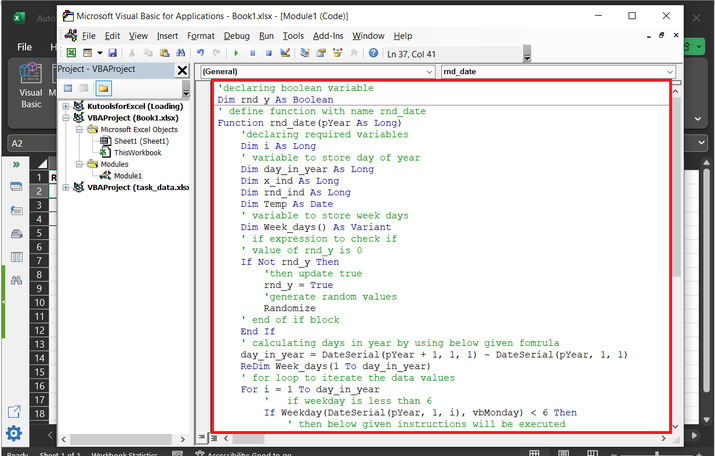### Step 7:

Press “Ctrl+S” to save the code, and after that open the sheet, to call the method “rnd_date(2023)” as pass year as a parameter value.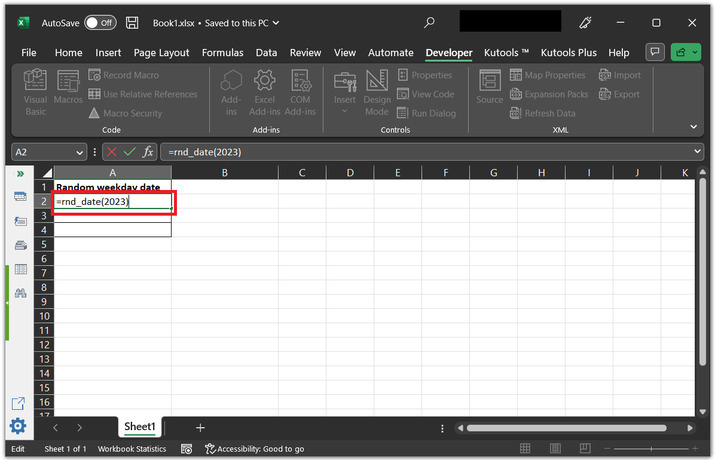### Step 8:

This will display random dates of the provided year. As, shown below: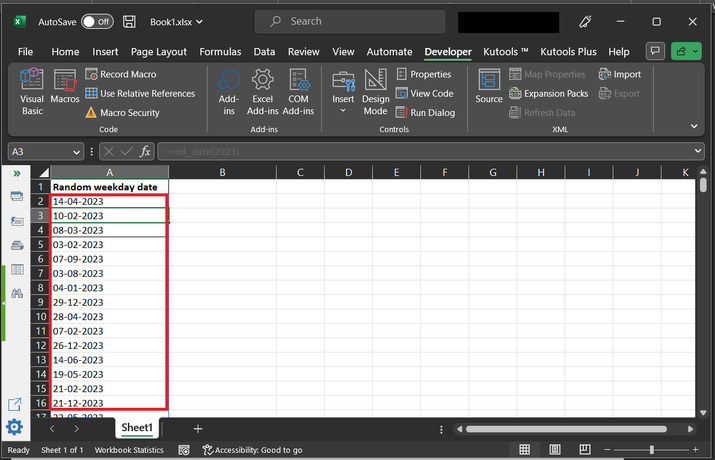## Conclusion:

This tutorial allow user to understand the way to generate the random weekday and weekend dates by using three different methods. After completing the tutorial user will able to easily generate the date.

Updated on: 17-Apr-2023

305 Views